# l Matrix Window

This window displays, and allows the editing of, the equilibrium geometry and vibrational modes for an electronic state. To bring up the window, right click on the state and select "l Matrix...". To display a particular normal mode, click on the appropriate column. Rx, Ry and Rz are the rotations around the x, y and z axes and Tx, Ty and Tz are the translations along the axes. They are calculated from the masses and equilibrium geometries and can't be edited.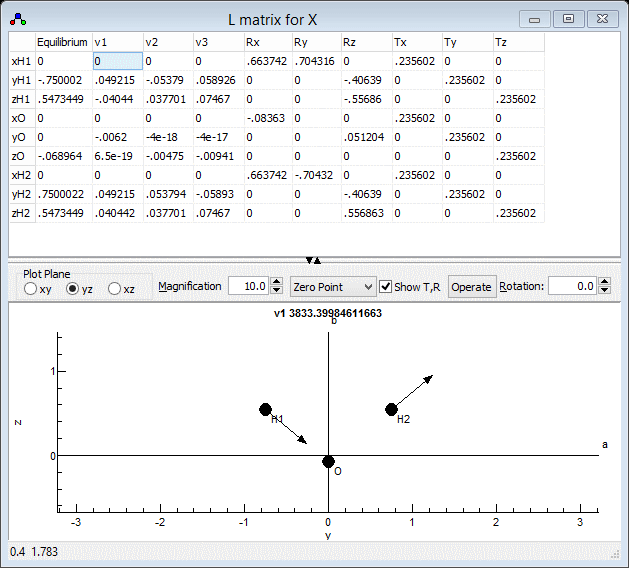The lower panel shows the atom positions, and a normal mode motion if a mode is selected. a, b, and c are the principal inertial axes, which are calculated from the given atomic positions.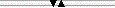Drag to resize the upper and lower parts.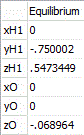Equilibrium positions of the atoms, in Å. These should be with respect to the centre of mass; if not see under the Operate button.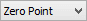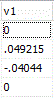Select matrix displayed and plotted in one of three forms: "Zero Point" is the displacements at the classical limits of the zero point motion. "adm (/10)" is the matrix M-½ l, and the values correspond to relative atomic displacements, and the units are 1/sqrt(atomic mass units). "l (/10)" is the l matrix; the values are dimensionless. In this form the matrix should be orthogonal; see under the Operate button for options to check and correct this.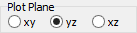Select plane to plot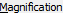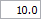This controls the length of the arrows plotted on each atom to show the motion of the atom in the selected normal mode. The number is the multiple of the zero point motion, assuming the motion is harmonic. If you can't see any arrows, you may need to increase the value set here.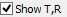Show Translational and Rotational normal modes. Note "Zero Point" does not make sense for these, so the values and plot are the same as "adm (/10)".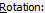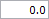Rotates the plotted image by the given angle about the horizontal axis.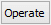This brings up a menu with the following options:
 Check Force update of the coordinates and l matrix from all other objects and plot. Print Force update of the coordinates and l matrix and show calculated state information in the log window Fix Centre of Mass Adjust atomic co-ordinates in the Nuclear co-ordinates objects so centre of mass is at the origin. Normalize Adjust the vibrational co-ordinates in Vibrational Modes objects to be correctly normalized. Orthogonalize Adjust vibrational co-ordinates in Vibrational Modes objects to be orthogonal and correctly normalized. Also fixes centre of mass if necessary.